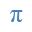Equation3-to-EquationGrindEQ Equation3-to-Equation converts old Equation Editor 3.x objects to Microsoft Equation format. Works with Microsoft Word for Windows, 32-bit and 64-bit compatible. Equation3-to-Equation is a part of MathType-to-Equation.

Update your old equations to new format;

Convert a whole Microsoft Word document or selected equations.

A new version of Microsoft Equation Editor was announced in 2007. This version is based on MathML, and includes many advantages in comparison with the previous one - Equation 3. Unfortunately, Equation 3 is not compatible with the new format, therefore old equations cannot be edited in the latest versions of Microsoft Word.

Fortunately, you can use GrindEQ Equation3-to-Equation converter, which will upgrade your old equations.

Equation3-to-Equation is a part of MathType-to-Equation module because Equation 3 and MathType were both created by Design Science, Inc., thus these equation editors are using similar formats. Thereby you can use MathType-to-Equation not only for converting MathType, but also for updating Equation 3 objects to the latest Microsoft Equation format.